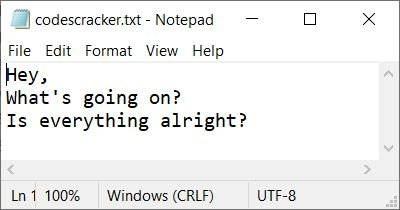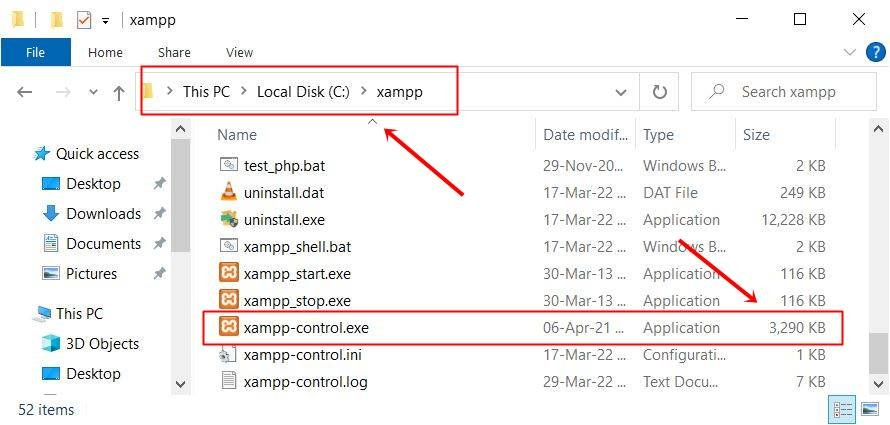# PHP filesize(): Get the size of the file

The PHP filesize() function returns the size of a specified file in bytes. For example:

```<?php
echo filesize("codescracker.txt");
?>```

The output produced by the above PHP example on the filesize() function is:And here is the snapshot of the file codescracker.txt, used in the above example:## PHP filesize() Syntax

The syntax of the filesize() function in PHP is:

`filesize(fileName)`

## PHP gets the file size in KB using filesize()

The following PHP code finds and prints the size of a file in terms of kilobytes (KB).

```<?php
\$myfile = "codescracker.txt";
\$x = filesize(\$myfile);
\$x = \$x/1024;

echo "<p>Size of File, \$myfile is \$x KB</p>";
?>```

The output produced by the above PHP example, to find and print the size of a file in KB, is:If you want to remove all the digits after the decimal, then put (int) before the size, in a similar way as done in the following program:

```<?php
\$myfile = "favicon.ico";
\$x = filesize(\$myfile);
\$x = (int)(\$x/1024);

echo "<p>Size = \$x KB</p>";
?>```

Now the output should be "Size = 30 KB," because the file favicon.ico is 30 KB. I've not used the codescracker.txt file in the example above, as the size of that file is 46 bytes, which will give 0 KB as output.

## PHP gets the file size in MB using filesize()

The following PHP code finds and prints the size of a file in terms of megabytes (MB).

```<?php
\$myfile = "C:/xampp/xampp-control.exe";
\$x = filesize(\$myfile);
\$x = (int)(\$x/1024/1024);

echo "<p>Size = \$x MB</p>";
?>```

The output produced by this PHP example, which prints the size of the xampp-control.exe file available in the C:/xampp/ directory, is shown in the snapshot given below:And here is the snapshot of the file along with the directory used in the above example:## PHP gets the file size in GB using filesize()

The following PHP code finds and prints the size of a file in terms of gigabytes (GB).

```<?php
\$myfile = "F:/sw/os/windows64.iso";
\$x = filesize(\$myfile);
\$x = (int)(\$x/1024/1024/1024);

echo "<p>Size = \$x GB</p>";
?>```

Since the size of file windows64.iso is 5.1GB, therefore after removing ".1," you will get Size = 5 GB as output.

### Advantages of the filesize() function in PHP

• The filesize() function is straightforward and only requires one line of code to determine the size of a file.
• The filesize() function is extremely efficient because it only retrieves the file's size rather than reading its entire contents.
• The filesize() function consumes little memory, making it appropriate for use with large files.
• The filesize() function accurately represents the file size, including any hidden characters or whitespace.

### Disadvantages of the filesize() function in PHP

• The filesize() function provides only the file's size and no other information regarding its contents.
• If the user controls the file being checked, the filesize() function poses a security risk. This may allow malicious users access to sensitive file information on the server.
• If the file cannot be read or if there are other problems with the file, the filesize() function does not provide detailed error messages.
• Depending on the platform, the filesize() function may behave differently on various platforms or operating systems, which may impact the accuracy of the file size measurement.

PHP Online Test

« Previous Tutorial Next Tutorial »